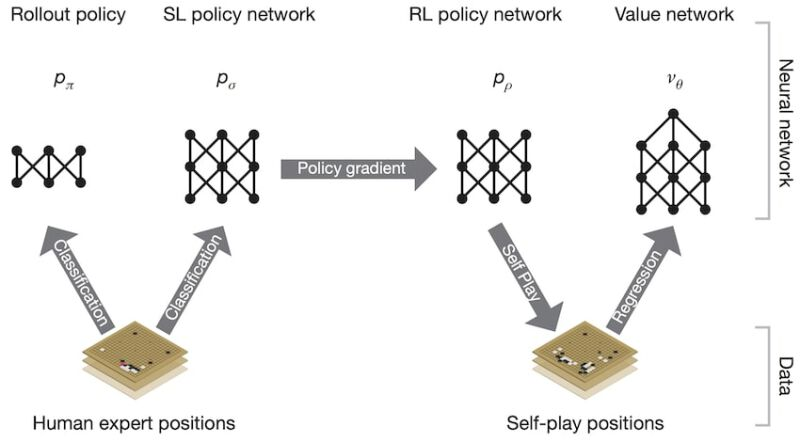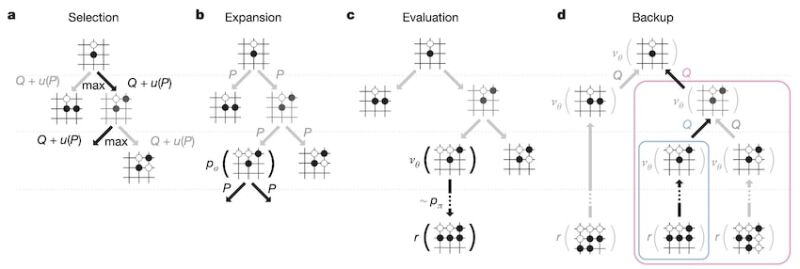Go’s game has long been considered the most challenging game of perfect information to implement in AI research due to its complexity. Usually, this type of game may be solved thanks to a search tree, which considers each possible move from a given state $$s$$ and computes a value for each one of them according to an optimal value function $$v^*(s)$$. That function gives, at every board position, the outcome of the game, i.e., the probability of winning with each particular disposition of the board. The problem with such a tree for Go is the complexity, as the number of possible moves to evaluate in each state is way too significant. As Demis Hassabis, CEO @DeepMind said:

“There are more possible Go positions than there are atoms in the universe.”

However, it is possible to reduce the size of the tree with two principles:

1. The tree’s depth can be reduced by position evaluation, which consists of truncating the tree at a specific state and evaluating its value with an approximate value function $$v(s) \approx v^*(s)$$.

2. The tree’s breadth can be reduced by sampling some possible moves according to a policy function $$p(a \vert s)$$ that gives a probability distribution over all possible moves $$a$$ in state $$s$$.

For AlphaGo, DeepMind introduces a new approach that uses deep neural networks for position evaluation (“value networks”) and for moves sampling (“policy networks”). These neural networks, combined with Monte Carlo simulation, represents the new search algorithm that created AlphaGo, the program that defeated the human world Go champion.

### How does AlphaGo work?

The neural networks were trained using a pipeline of several machine learning algorithms. The search algorithm then combines the policy and value networks in a Monte Carlo Tree Search (MCTS) algorithm.##### A. Supervised learning of policy networks

The first stage of the training pipeline consists of training a supervised learning (SL) policy network $$p_{\sigma}$$ directly from expert human moves. The weights $$\sigma$$ are trained in a 13-layer neural network on randomly sampled state-action pairs from a dataset of 30 million positions collected from the KGS Go Server, using stochastic gradient ascent to maximize the likelihood of a human move to be selected in a given state. The network takes as input a representation of the board state and outputs a probability distribution over all legal moves. The SL policy network provides fast and efficient learning updates with immediate feedback and high-quality gradients.

Besides, a faster but less accurate rollout policy $$p_{\pi}$$ is trained using a linear softmax of small pattern features. This rollout policy is used for fast node evaluation in the search algorithm.

##### B. Reinforcement learning of policy networks

The second stage of the training pipeline consists of training a reinforcement learning (RL) policy network $$p_{\rho}$$ that improves the SL policy network by self-playing. However, to avoid overfitting that could happen if all games were played between the current policy network and itself, the games are played between the current policy network $$p_{\rho}$$ and a randomly selected previous iteration of itself, in that way stabilizing the training.

The weights $$\rho$$, initially set to $$\sigma$$, are trained using stochastic gradient ascent to optimize the outcome of self-play games. That way, the policy will adjust towards the correct goal of winning games, rather than maximizing predictive accuracy.

##### C. Reinforcement learning of value networks

The final stage of the training pipeline consists of estimating a value function $$v^{p_{\rho}}(s)$$ that predicts the outcome of the game from state $$s$$ of all games played by the RL policy network $$p_{\rho}$$ against itself. This value function is approximated using a value network $$v_{\theta}(s) \approx v^{p_{\rho}(s)}$$. The weights $$\theta$$ are trained by regression on state-outcome pairs, using stochastic gradient descent to minimize the mean squared error (MSE) between the predicted value and the corresponding outcome.

A new dataset of uncorrelated self-play positions was generated to avoid overfitting to the strongly correlated positions within games, consisting of 30 million distinct positions, each sampled from a different game.

##### D. Searching with policy and value networksSelection. The first in-tree phase consists in selecting an action for each node between the root and a leaf, so as to maximize action value $$Q(s,a)$$ plus a bonus $$u(s_t,a)$$ that is proportional to the prior probability $$P(s,a)$$ but decays with the visit count $$N(s,a)$$ in order to encourage exploration: $$a_t = \text{argmax}_a (Q(s_t,a) + u(s_t,a))$$

Expansion. When the simulation reaches a leaf node $$s_L$$ for which the visit count exceeds a threshold, the leaf may be expanded to a new node that is processed once by the SL policy network $$p_{\sigma}$$. The output probabilities are then stored as prior probabilities $$P$$ for each legal action.

Evaluation. The next in-tree phase consists in evaluating the leaf node $$s_L$$ as a weighted average of parameter $$\lambda$$ that mixes together the value network $$v_{\theta}(s_L)$$ and the outcome $$z_L$$ of a random rollout played out until a terminal step using the fast rollout policy $$p_{\pi}$$: $$V(s_L) = (1-\lambda) v_{\theta}(s_L) + \lambda z_L$$

Backup. The final in-tree phase consists of updating the action values and visit counts of all traversed edges of the tree by a backward pass. Once the search is complete, each edge accumulates the visit count and the mean evaluation of all simulations passing through it, and the algorithm chooses the most visited move from the root.

### How good is AlphaGo?

Go programs can be evaluated by running an internal tournament and measuring the Elo rating of each program. All Go programs were executed on a single machine with identical specifications and received a maximum of 5 seconds of computation time per move.

The tournament results showed that AlphaGo is significantly stronger than any previous Go programs, winning 494 out of 495 games. To go even further, AlphaGo also played games with four handicap stones, i.e., free moves for the opponent, and won more than 75% of these games against the strongest Go programs (Crazy Stone, Zen, and Pachi).

To prove their value network’s efficiency for position evaluation, they also played games with variants of AlphaGo where the position evaluation only used this value network ($$\lambda = 0$$). Even in that case, AlphaGo exceeded all other Go programs’ performance, even though a mixed evaluation ($$\lambda = 0.5$$) performed best.

Finally, a distributed version of AlphaGo exploiting multiple machines, that won 77% of the games against single-machine AlphaGo and 100% of its games against other Go programs, was evaluated against European Go champion of 2013 to 2015, Fan Hui, in a formal five-game match. At the end of the match, AlphaGo was the first computer Go program to defeat a human professional player, with a 5-0.

### Summary

This work aimed to demonstrate the feats of artificial intelligence by addressing one of the most complex two-players strategy games: Go. The Google DeepMind team developed a Go program that plays at the level of the strongest human players; a feat previously thought to be at least one decade away. Go was a grand challenge for artificial intelligence because of its challenging decision-making task and intractable search space that it appears infeasible to make a direct approximation using a policy or value function.

However, DeepMind developed, for the first time, effective move selection and position evaluation functions using deep convolutional neural networks trained by a novel combination of supervised and reinforcement learning. These neural networks were combined with Monte Carlo rollouts in a high-performance tree search engine.

By achieving to reach a professional level in Go, AlphaGo has provided confidence that human-level performance can now be accomplished in other seemingly intractable artificial intelligence domains.